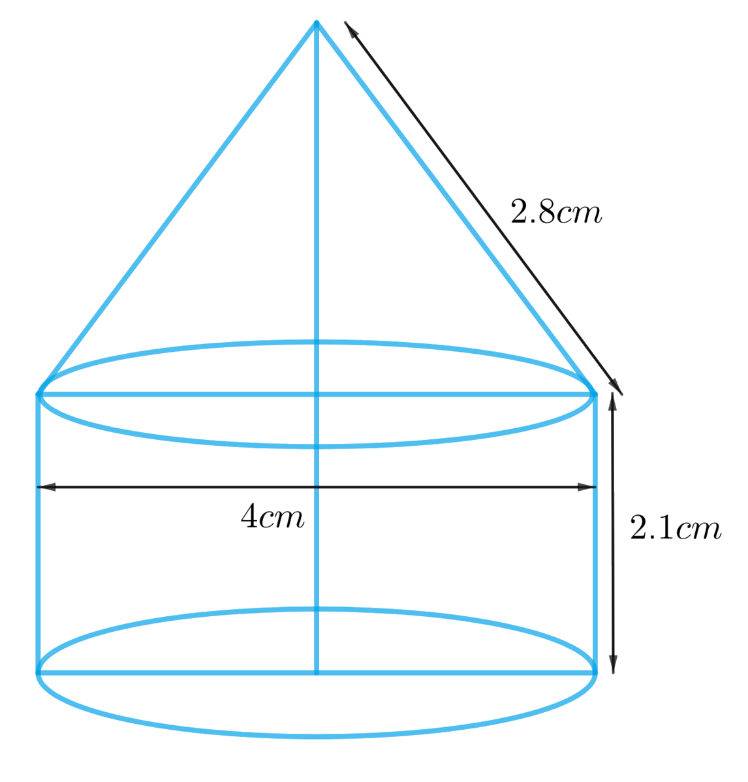# Ex.13.1 Q7 Surface Areas and Volumes Solution - NCERT Maths Class 10

Go back to  'Ex.13.1'

## Question

A tent is in the shape of a cylinder surmounted by a conical top. If the height and diameter of the cylindrical part are $$2.1\,\rm{ m}$$ and $$4 \,\rm{m}$$ respectively, and the slant height of the top is $$2.8 \,\rm{m}$$, find the area of the canvas used for making the tent. Also, find the cost of the canvas of the tent at the rate of Rs $$500 \;\rm{per}\, \rm{m}^2$$. (Note that the base of the tent will not be covered with canvas.)

## Text Solution

What is  known?

The height and diameter of the cylindrical part are $$2.1 \rm m$$ and $$4 \rm m$$ respectively, and the slant height of the conical top is $$2.8 \rm m$$. The rate of the canvas of the tent is $$₹ 500$$ per $$\rm m^2$$.

What is unknown?

Area and cost of the canvas used for making the tent

Reasoning:

We can create the figure of the tent as per given informationFrom the figure it’s clear that, diameter of the cylindrical part is equal to the diameter of the conical part as the cylindrical part is surmounted by the conical part.

Since its given that the base of the tent will not be covered by the canvas then the base of the cylindrical part of the tent is not included to get the area of the canvas required.

Visually, the surface of the tent includes the curved surface of the cylindrical part and curved surface of the conical part only.
Area of the canvas used for making the tent is the surface area of the tent.

Area of the canvas used $$=$$ CSA of the cylindrical part $$+$$ CSA of the conical part

We will find the area of the canvas by using formulae;
CSA of the cone $$= \pi rl$$
where $$r$$ and $$l$$ are radius and slant height of the cone respectively.
CSA of the cylinder  $$= 2\pi rh$$
where $$r$$ and $$h$$ are radius and height of the cylinder respectively.

Cost of the canvas of the tent $$=$$ Area of the canvas $$\times$$ Rate of the canvas

Steps:

Given

Height of the cylindrical part $$h$$ $$= 2.1 \,\rm{m}$$

Diameter of the cylindrical part, $$d= 4 \,\rm{m}$$

Radius of the cylindrical, \begin{align}r = \frac{d}{2} = \frac{{4 \rm m}}{2} = 2 \rm m\end{align}

Radius of the cone,$$r = 2 \rm m$$
Slant height of the cone,  $$l = 2.8 \rm m$$

Area of the canvas used $$=$$ CSA of the cylindrical part $$+$$ CSA of the conical part

\begin{align}&= 2\pi rh + \pi rl\\&= \pi r\left( {2h + l} \right)\\&= \frac{{22}}{7}\! \times\! 2 \rm m \!\times\! ( 2 \!\times\! 2.1 \rm m \!+\! 2.8 \rm m) \\&= \frac{{44}}{7} \rm m \times 7m\\&= 44 \rm {m^2}\end{align}

Cost of the canvas of the tent $$=$$ Area of the canvas $$\times$$ Rate of the canvas

\begin{align}&= 44 \rm {m^2} \times ₹\,500/{m^2}\\&= ₹ 22000\end{align}

Learn from the best math teachers and top your exams

• Live one on one classroom and doubt clearing
• Practice worksheets in and after class for conceptual clarity
• Personalized curriculum to keep up with school